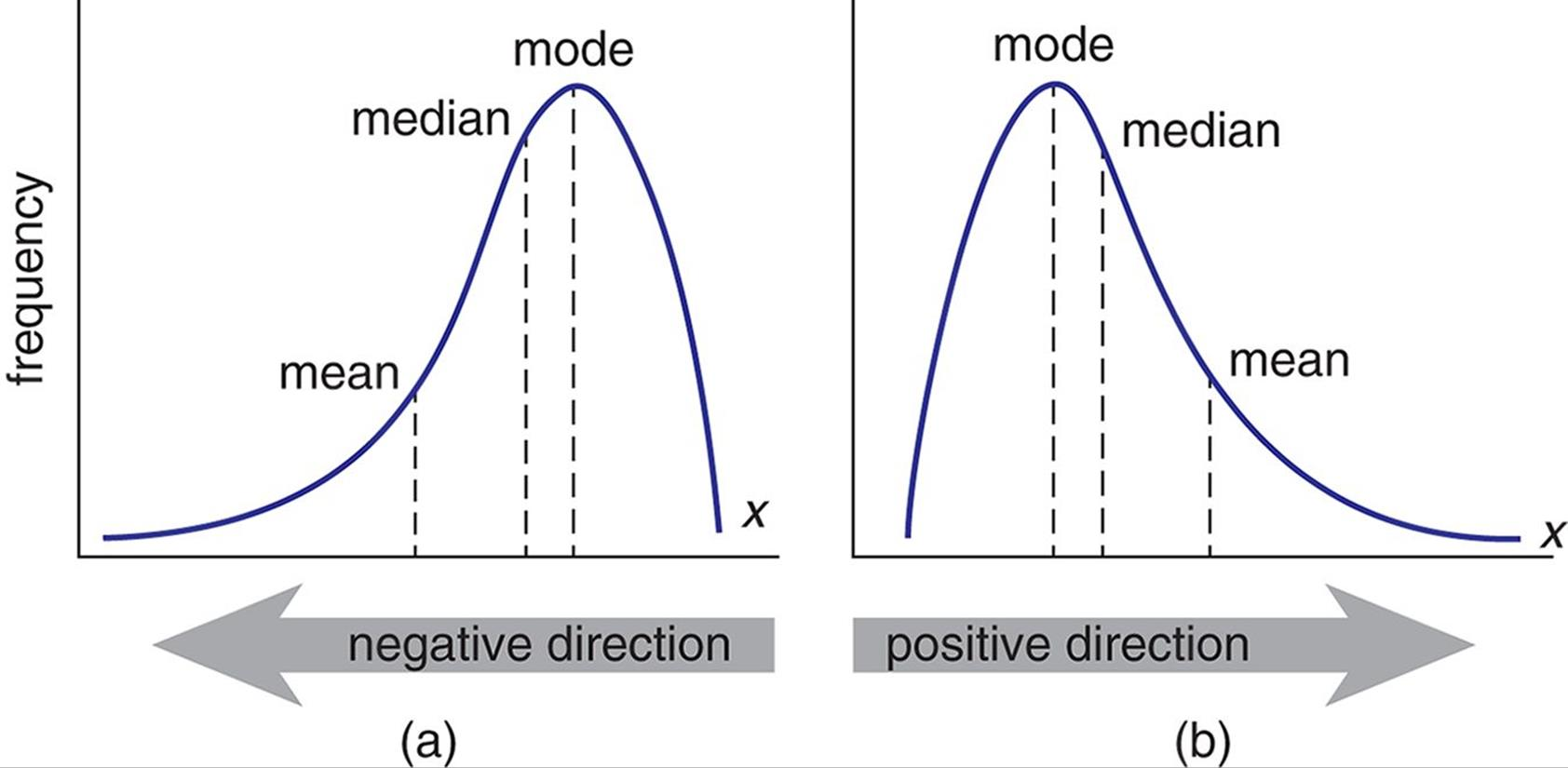/  Top Machine learning interview questions and answers   /  What are the differences between Mean, Median, Mode? How these are helpful to deal with the missing values in the given dataset?## What are the differences between Mean, Median, Mode? How these are helpful to deal with the missing values in the given dataset?

Mean

Mean is the average of the Dataset. It is the ratio of Sum of total observations to the Total number of observations.Median

It is the middle value of the Dataset. If the total number of observations in the Dataset is odd in number, then median is the middle most value or observation. If the total number of observations in the dataset are even in number, then the median is given by the average of the middle two values of the dataset.Mode

Mode is the most frequently occurred observation or value in the entire Dataset.

If the data is Normally distributed then, missing values can be imputed or replaced by mean of the all observations of the dataset.

If the data is skewed then, it is better to impute or replace the missing values by Median of all observations of the Dataset.

When the Data is related to the frequency, then mode is used to replace or impute the missing values in the respective Dataset.